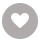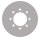# Data Analysis with Stata电子书

82人正在读 | 0人评论9.8

• 读书简介
• 目录
• 累计评论(0条)
• 读书简介
• 目录
• 累计评论(0条)
Explore the big data field and learn how to perform data analytics and predictive modelling in STATAAbout This BookVisualize and analyse data in STATA to devise a business strategyLearn STATA programming and predictive modelingDiscover how you can become a data scientist with the power of STATA Who This Book Is For This book is for all the professionals and students who want to learn STATA programming and apply predictive modelling concepts. This book is also very helpful for experienced STATA programmers as it provides advanced statistical modelling concepts and their application.What You Will LearnPerform important statistical tests to become a STATA data scientistBe guided through how to program in STATAImplement logistic and linear regression modelsVisualize and program the data in STATAAnalyse survey data, time series data, and survival dataPerform database management in STATA In Detail STATA is an integrated software package that provides you with everything you need for data analysis, data management, and graphics. STATA also provides you with a platform to efficiently perform simulation, regression analysis (linear and multiple) [and custom programming. This book covers data management, graphs visualization, and programming in STATA. Starting with an introduction to STATA and data analytics you’ll move on to STATA programming and data management. Next, the book takes you through data visualization and all the important statistical tests in STATA. Linear and logistic regression in STATA is also covered. As you progress through the book, you will explore a few analyses, including the survey analysis, time series analysis, and survival analysis in STATA. You’ll also discover different types of statistical modelling techniques and learn how to implement these techniques in STATA.Style and approach This book is a hands-onguide to STATA programming and statistical modelling providing many STATA code examples and taking. You through the working of the code in detail.

Data Analysis with Stata

Data Analysis with Stata

Credits

www.PacktPub.com

Support files, eBooks, discount offers, and more

Why subscribe?

Free access for Packt account holders

Instant updates on new Packt books

Preface

What this book covers

What you need for this book

Who this book is for

Conventions

Customer support

Errata

Piracy

Questions

1. Introduction to Stata and Data Analytics

Introducing data analytics

The Stata interface

Data-storing techniques in Stata

Directories and folders in Stata

Insheet

Infix

The Stat/Transfer program

Manual typing or copy and paste

Variables and data types

Indicators or data variables

Examining the data

How to subset the data file using IN and IF

Summary

2. Stata Programming and Data Management

The labeling of data, variables, and variable transformations

Summarizing the data and preparing tabulated reports

Appending and merging the files for data management

Macros

Loops in Stata

While loops

Summary

3. Data Visualization

Scatter plots

Line plots

Histograms and other charts

Box plots

Pie charts

Pyramidal graphs

Vio plots

Ci plots

Statistical calculations in graphs

Curve fitting in Stata

Summary

4. Important Statistical Tests in Stata

T tests

Two independent sample t tests

The chi-square goodness of fit test

ANOVA

One-way repeated ANOVA measures

MANOVA

Fisher's exact test

The Wilcoxon-Mann-Whitney test

Summary

5. Linear Regression in Stata

Linear regression

Linear regression code in Stata

Variance inflation factor and multicollinearity

Homoscedasticity

Summary

6. Logistic Regression in Stata

The logistic regression concept

Logit

Logistic regression in Stata

Logistic regression for finance (loans and credit cards)

Summary

7. Survey Analysis in Stata

Survey analysis concepts

Survey analysis in Stata code

Cluster sampling

Summary

8. Time Series Analysis in Stata

Time series analysis concepts

Code for time series analysis in Stata

Summary

9. Survival Analysis in Stata

Survival analysis concepts

Applications and code in Stata for survival analysis

Building a model

Proportionality assumption

Summary

Index

01

### Java 9 Dependency Injection￥67.99

Krunal Patel,Nilang Patel
￥67.99
02

Pedro Teixeira
￥91.99
03

Trevor James
￥91.99
04

Rohit Rai
￥76.99
05

John Farrar
￥91.99
06

Faruk Akgul
￥76.99
07

Jose A. Tizon
￥137.99
08

John Arundel
￥137.99
09

Tochi Eke-Okoro
￥70.99
10

### Clojure Data Analysis Cookbook￥167.99

Eric Rochester
￥167.99
•关注我们

最受欢迎的阅读产品

关注我们：

•关于我们

欢迎反馈宝贵意见给我们

客服书吧：当当云阅读问答